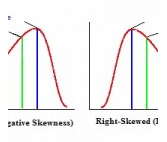Kelly’s Measure of Skewness, Business Mathematics & Statistics

# Kelly’s Measure of Skewness, Business Mathematics & Statistics - Business Mathematics and Statistics - B Com

## What is Kelly’s Measure of Skewness?

A left-skewed distribution.

Kelly’s Measure of Skewness is one of several ways to measure skewness in a data distribution. Bowley’s skewness is based on the middle 50 percent of the observations in a data set. It leaves 25 percent of the observations in each tail of the distribution. Kelly suggested that leaving out fifty percent of data to calculate skewness was too extreme. He created a measure to find skewness with more data. Kelly’s measure is based on P90 (the 90th percentile) and P10 (the 10th percentile). Only twenty percent of observations (ten percent in each tail) are excluded from the measure.

## Kelly’s Measure Formula.

Kelley’s measure of skewness is given in terms of percentiles and deciles(D). Kelley’s absolute measure of skewness (Sk)is:

Sk=P90 + P10 – 2*P50 = D9 + D1-2*D5.

This formula is not practically used. In fact, Kelly’s measure of skewness is rarely used at all, even in it’s more common form, which is measured as coefficient of skewness:

SP = P90 – 2*P50 + P10 / P90 – P10

What does Kelly’s Measure of Skewness Tell us?

elly’s Measure of Skewness gives you the same information about skewness as the other three types of skewness measures (Bowley skewness, Pearson Mode Skewness and Momental Skewness.).

A measure of skewness = 0 means that the distribution is symmetrical.
A measure of skewness > 0 means a positive skewness.
A measure of skewness < means a negative skewness.

If you prefer an online interactive environment to learn R and statistics, this free R Tutorial by Datacamp is a great way to get started. If you're are somewhat comfortable with R and are interested in going deeper into Statistics, try this Statistics with R track.The document Kelly’s Measure of Skewness, Business Mathematics & Statistics | Business Mathematics and Statistics - B Com is a part of the B Com Course Business Mathematics and Statistics.
All you need of B Com at this link: B Com

115 videos|142 docs

## FAQs on Kelly’s Measure of Skewness, Business Mathematics & Statistics - Business Mathematics and Statistics - B Com

 1. What is Kelly's Measure of Skewness?Kelly's Measure of Skewness is a statistical measure used to determine the asymmetry or lack of symmetry in a distribution. It quantifies the degree to which a dataset deviates from a perfectly symmetrical distribution. It is a dimensionless measure and can be positive, negative, or zero, indicating different types of skewness.
 2. How is Kelly's Measure of Skewness calculated?Kelly's Measure of Skewness is calculated using the formula: Skewness = (Mean - Mode) / Standard Deviation. The numerator represents the difference between the mean and mode of the dataset, while the denominator represents the standard deviation. By dividing the difference by the standard deviation, we obtain a normalized measure of skewness.
 3. What does a positive skewness value indicate?A positive skewness value indicates a right-skewed distribution. In a right-skewed distribution, the tail on the right side is longer or fatter than the left side. This means that there are more extreme values on the right side, which pulls the mean towards the higher values. The mode is usually lower than the mean in a right-skewed distribution.
 4. What does a negative skewness value indicate?A negative skewness value indicates a left-skewed distribution. In a left-skewed distribution, the tail on the left side is longer or fatter than the right side. This means that there are more extreme values on the left side, which pulls the mean towards the lower values. The mode is usually higher than the mean in a left-skewed distribution.
 5. What does a skewness value of zero indicate?A skewness value of zero indicates a perfectly symmetrical distribution. In a symmetrical distribution, the mean, median, and mode are all equal. The data is evenly distributed around the center, and there are an equal number of extreme values on both sides. This means that there is no skewness present in the distribution.

115 videos|142 docsExplore Courses for B Com examSignup to see your scores go up within 7 days! Learn & Practice with 1000+ FREE Notes, Videos & Tests.
10M+ students study on EduRev
Track your progress, build streaks, highlight & save important lessons and more!
Related Searches

,

,

,

,

,

,

,

,

,

,

,

,

,

,

,

,

,

,

,

,

,

,

,

,

;